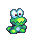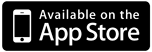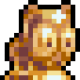#Frogatto & Friends is an action-adventure game, starring a certain quixotic frog. Give it a try!
We're trying to push 2D platforming, pixel-art, and music into uncharted territory. We hope you like the results!
Also, Frogatto has a very flexible game engine you can use to make your own creations.

## A Gentle Introduction to Frogatto Formula Language

May 25th, 2012 by Sirp

Frogatto Formula Language (FFL) is the language used to customize Frogatto and control its behavior. I’ve talked about how Frogatto’s event handling system works before, right here.

Today I’m going to talk more about how FFL works, giving an overview of the language.

### Getting Started

Becoming familiar with FFL is easy. Just start Frogatto and press ctrl+d to enter the debug console. The debug console allows you to type FFL statements and have them evaluated right then and there. Try some basic commands:

``` --> 4 + 5 9 --> 7*3 + 9 30 --> 7*(3 + 9) 84 --> ```

### Accessing an Object

When an FFL formula is executed, it has an object it is operating on. When you first open the debug console, that object is the player. Let’s try some basic formulas that find information about the player:

``` --> x 416 --> y 383 --> type 'frogatto_playable' --> animation 'stand' --> ```You can output the entire player object by entering `self` as a command — it will show you all the fields the player has to offer!

### Where Clauses

For convenience, FFL supports where clauses that appear at the end of an expression to define additional values:

``` --> 2*n + n*n where n = 5 35 ```

### Object Mutation

So far we’ve seen how to query the values from an object. If you enter a formula that returns a command, the debug console will execute the command on the object. It might be a good idea to pause the game, by pressing ctrl+p before executing commands, so you can clearly see what’s going on.

Let’s try executing some commands:

``` --> set(y, y-250) (0xaebeb30) {}\ --> set(facing, 1) (0xaebec80) {} --> set(upside_down, true) (0xafbed90) {} --> ```Remember, what you are executing here in the debug console is the same kind of thing you would execute in an event handler when it is executed.

When the debug console is open, you can click on objects to select them, and they will become the focused object — they will be the context of any formulas you enter. For instance, you could click on a door, and then start typing commands to modify it.

### Basic data types

As we’ve seen, Frogatto can handle manipulation of integers. You may have noticed it also supports strings. e.g.

``` --> type 'frogatto_playable' ```

Strings can be concatenated, just as integers can be added:

``` --> 'abc' + 'def' 'abcdef' ```

You can also multiply a string by an integer:

``` --> 'abc' * 4 'abcabcabcabc' ```

Other types, such as integers, can be concatenated to a string using +:

``` --> 'you have ' + 5 + ' coins' 'you have 5 coins' ```

FFL also supports decimal numbers:

``` --> 4.25 * 0.3 1.275 --> 10.8 + 5 15.8 --> -0.5 - 0.8 -1.3 ```

FFL decimals are not floating point numbers. They use fixed point arithmetic and store up to six decimal places. Their behavior is consistent across machines.

FFL also supports the boolean types, true and false as well as the value null to represent no object.

### Basic Operators

FFL supports the following basic operators:

• + – * /: arithmetic operations
• and or not: boolean operators
• %: modulo operator. e.g. `9%8 -> 1`
• ^: exponentiation operator. e.g. `2^8 -> 256`
• = != <>=: comparison operators

Note that unlike many languages, = is used to test for equality, as FFL doesn’t have built-in assignment. Assignment is done by returning a set() object from a formula, requesting the game engine to perform the equivalent of an assignment.

### Random Numbers

FFL supports random numbers using the `d` operator:

``` --> 3d6 5 --> 3d6 11 --> 3d6 12 --> 50d100 2613 --> 3d faces where faces = 10 12 --> ndice d faces where ndice = 3, faces = 6 16 ```

### Functions

FFL has a wide range of built-in functions. A function is executed using function_name(parameters). A fundamental function is the if function:

``` --> if(2 < 4, 1, 2) 1 --> if(2 > 4, 1, 2) 2 ```

if tests the first parameter and if it’s true, it will return the second parameter, otherwise it will return the third parameter. The third argument is optional, and will be null if not provided.

``` --> if(1 > 2, 5) null ```

There are also functions to convert values from one type to another, or test the type of a value:

``` --> str(4) '4' --> is_int(4.2) false --> is_int(4) true --> is_string('abc') true --> is_string(4) false --> floor(4.2) 4 --> ceil(4.2) 5 --> round(4.2) 4 ```

### Lists

FFL supports lists which are enclosed in square brackets:

``` --> [5, 2+4] [5, 6] ```

Lists can be appended using the + operator:

``` --> [3, 5] +  [3, 5, 7] --> ['hello', 'there'] + [5, true, [4, 5]] ['hello', 'there', 5, true, [4, 5]] ```

Note how lists can be nested. i.e. you can have lists of lists. Lists can be multiplied just like strings:

``` --> [1, 2, 3] * 3 [1, 2, 3, 1, 2, 3, 1, 2, 3] ```

A single element of a list can be accessed using square brackets:

``` --> a where a = [1,2,3,4] 3 ```

You can also take a slice of a list using [begin:end] after the list:

``` --> a[2:5] where a = [1,2,3,4,5,6,7] [3,4,5] --> a[1:] where a = [1,2,3,4,5,6,7] [2,3,4,5,6,7] ```

You can find the number of elements in a list using the size() function:

``` --> size(a) where a = [1,2,3] 3 ```

Lists can be compared using = and != operators, and also using the relational operators,=. For instance,

``` --> [2,3,4] < [5,6] true --> [2,3,5] false ```

You can find if an item is in a list using the `in` operator:

``` --> 3 in [2,3,4] true ```

### Functions for Operating on Lists

FFL supplies a range of functions for operating on lists. One such function is the map function:

``` map(list, expression): evaluates expression once for each item in list, giving 'value' as the name of the item. ```

This is perhaps best explained with an example:

``` --> map([1,2,3,4], value*value) [1, 4, 9, 16] ```

Note how the expression `value*value` is evaluated once for each item in the list, with value containing that item. One thing that might seem strange to experienced programmers is how the parameter passed to the function is not eagerly evaluated, but rather, the function chooses when to evaluate it, and even what context to evaluate it in.

The map function can take an additional argument specifying the name of the parameter, to something other than value. For instance,

``` --> map([1,2,3,4], n, n*n) [1, 4, 9, 16] ```

The range function is useful for generating a list of integers:

``` --> range(10) [0, 1, 2, 3, 4,5, 6, 7, 8, 9] --> range(5, 10) [5, 6, 7, 8, 9] ```

The range function is often useful to produce a list to pass to map.

The filter function is useful for taking certain elements of a map that pass a certain criteria:

``` filter(list, expression): evaluates expression once for each item in list, and returns a list of all the items for which the expression was true. ```

For instance,

``` --> filter(range(10), value%2 = 1) [1, 3, 5, 7, 9] ```

### Map + Filter + Range: Example

The spawn function can be used to create an object. For instance, `spawn('coin_silver', x, y-80, 1)` will create a silver coin 80 pixels above Frogatto’s head. Let’s suppose we wanted to create ten coins in a row, we could do this:

``` --> map(range(10), spawn('coin_silver', (x - 200) + value*30, y - 80, 1)) ```

This will create a list of 10 spawn commands, creating 10 coins spaced out above Frogatto’s head.Now suppose we wanted to move all these coins up by 20 pixels. `level.chars` holds a list of all the objects in the level. We could filter by type to find all the coins in the level, and then use map to map these objects to set commands:

``` --> map(filter(level.chars, value.type = 'coin_silver'), set(value.y, value.y - 10)) ```

This will move all silver coins in the level up 10 pixels.

### List Comprehensions

Frogatto supports a convenient way to build lists, known as list comprehensions. Let’s start with an example:

``` [n^2 | n <- range(10)] = [0, 1, 4, 9, 16, 25, 36, 49, 64, 81] ```

This is equivalent to saying "for each n in range(10), generate a list with n^2". A list comprehension can also contain filtering conditions on the input list. For instance, if we wanted to square only even numbers, we could do this:

``` [n^2 | n <- range(10), not n%2] = [0, 4, 16, 36, 64] ```

A list comprehension can take multiple input lists, in which all the combinations of elements in each list are used. For instance:

``` [a + ' ' + b | a <- ['small', 'big', 'huge'], b <- ['ant', 'bird', 'bat']] = ['small ant', 'big ant', 'huge ant', 'small bird', 'big bird', 'huge bird', 'small bat', 'big bat', huge bat'] ```

List comprehensions are a convenient alternative to using map and filter. This formula from earlier can be re-written using list comprehensions:

``` map(range(10), spawn('coin_silver', (x - 200) + value*30, y - 80, 1)) ```

As a list comprehension:

``` [spawn('coin_silver', (x - 200) + n*30, y - 80, 1) | n <- range(10)] ```

### Dictionaries

FFL supports dictionaries, also known as maps. A dictionary is a series of key:value pairs written within curly brackets. For instance,

``` --> {y: 5, x: 2} {'x': 2, 'y': 5} ```

Note how the keys are assumed to be strings, rather than x and y being interpreted as expressions. Also, keys in a map are always sorted.

Any FFL type may be a key in a map. Integers, booleans, lists, and even other maps are valid keys:

``` --> {[3,4,5]: 7, {a: 5}: 9} {[3, 4, 5]: 7, {'a': 5}: 9} ```

Elements in maps can be looked up either using the . operator, or using []:

``` --> a.name where a = {name: 'Frogatto'} 'Frogatto' --> a['name'] where a = {name: 'Frogatto'} 'Frogatto' ```

The . operator only works on string keys that are valid identifiers (a valid FFL identifier begins with a letter or underscore and consists of letters, underscores, and digits). The [] operator can be used for all types of keys.

### Objects

FFL supports objects as data types. An object looks and behaves similar to a dictionary, however an object may have additional behavior or properties to a regular dictionary. FFL doesn't have support for directly creating objects, but an FFL formula might be given access to an object, or have an object returned by a function.

One example of an object is the game object that represents Frogatto. When you start the debugger, you have access to the player's game object. That is why you can enter a formula such as `x` or `y` or `type` and see a result. The level itself is exposed as an object, and all objects have access to it through their `level` property.

Another example of objects are command objects. Command objects are created and returned when you call a function like set() or spawn(). These types of objects don't actually have any interesting values that can be queried from FFL. That is why you see a result like this:

``` --> spawn('coin_silver', 10, 10, 1) (0xb825640){} ```

This formula returns a spawn command object, but the contents of the object are opaque to FFL, so all FFL knows about it are the address and then has an empty {} properties map. FFL doesn't need to know how to query or manipulate a command object though, since the entire idea is to return it to the game engine, and the game engine knows how to execute the command and make the object get spawned.

This is a general tutorial on FFL, so I'm not going to get too into the different types of objects available. The crucial thing to understand, though, is that FFL is a pluggable language that can be used in different contexts. The most common use for FFL right now is to write Frogatto event handlers with, however it's designed to be used in different contexts -- we also use it to write editor scripts with, gui scripts, and scripts to manipulate FSON documents. Depending on how and where you use FFL you will have access to different types of objects.

### Functions

You can define your own functions in FFL:

``` --> def f(n) n^2 + 5; f(5) + 2 32 ```

Functions are themselves variables that can be passed to functions and otherwise treated as regular values. For instance,

``` --> def f(fn, x) fn(5, x); def add(a, b) a + b; f(add, 2) 7 ```

Note: Built-in functions currently cannot be treated as values or passed around. We are looking to fix that in a future release.

Functions can have default arguments specified:

``` --> def f(a, b=5) a+b; [f(1,1), f(2)] [2, 7] ```

### Recursion

Functions can call themselves. As an example, suppose you wanted to write a function `index(list, item) -> returns the index of the first occurrence of item in list, or -1 if item is not in list`

you could do it like this:

``` def index(list, item, n=0) if(list = [], -1, if(list = item, n, index(list[1:], item, n+1))) ```

As another example, FFL has a builtin sort() function, but if you wanted to make your own sort, you could implement quicksort like this:

``` def qsort(list) if(size(list) qsort(filter(list, value < pivot)) + filter(list, value = pivot) + qsort(filter(list, value > pivot)) where pivot = list) ```

### True or False?

At certain times, FFL will test whether a value is considered 'true' or 'false'. The following values are considered false:

• false
• null
• 0
• 0.0
• []
• {}

All other values are considered true. Note that the and and or operators have particularly interesting behavior: or will evaluate to its first argument if its first argument is a true value, otherwise it will evaluate to its second argument. Likewise, and will evaluate to its first argument if its first argument is a false value, otherwise it will evaluate to it second argument.

``` --> 5 or 2 5 --> false or 8 8 ```

### 8 responses to “A Gentle Introduction to Frogatto Formula Language”

1.Aaron says:

Hey this game seems nicely programmed, but I blew all my money a week ago, what with all the new releases. Can I get a promocode? thanks.

2.Aaron says:

email me at aaron.99.chen@gmail.com

3.fenghelong says:

Hello,I read the program of the FFL but I can not understand the part of recursion,can you help me?Give me some other information?

4.Sirp says:

fenghelong: Recursion is a common feature in many languages, and you can read about it on wikipedia, which will have many resources.

However, as far as the Frogatto engine is concerned, recursion is a rather advanced feature. I’d recommend playing around with the rest of the language and seeing what you can make.

Recursion is actually hardly ever needed for most things you’d want to do with the Frogatto engine.

6.DDR says:
Note: The syntax for list comprehensions has been changed to, eg, ```[x*y | x <- [1,2,3], y <- [10,20,30]]```. This would render the list `[10, 20, 30, 20, 40, 60, 30, 60, 90]`.